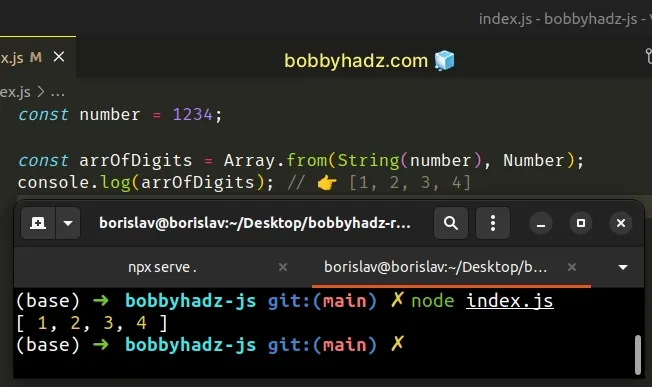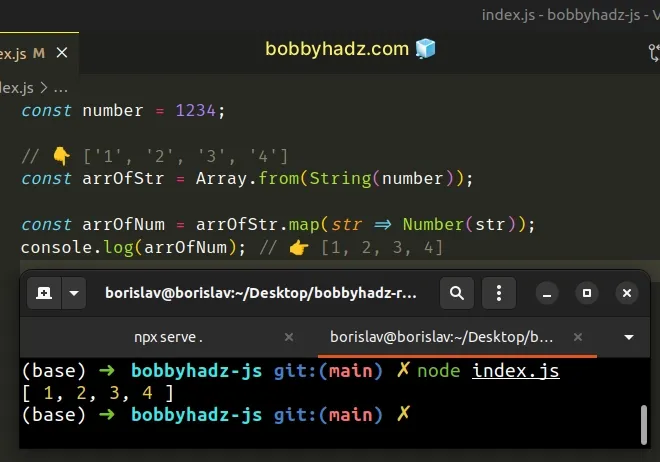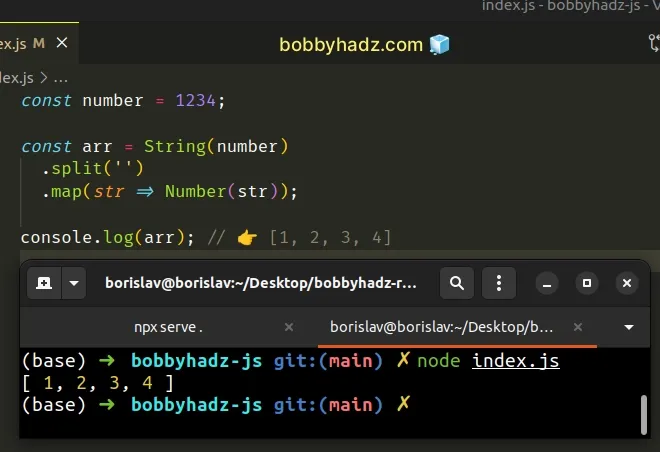# Split a Number into an Array in JavaScriptLast updated: Dec 31, 2022
3 min## #Split a Number into an Array in JavaScript

To split a number into an array:

1. Use the `String()` constructor to convert the number to a string.
2. Use the `Array.from()` method to convert the string to an array of digits.
3. Use the `map()` method to convert each string in the array to a number.
index.js
```Copied!```const number = 1234;

const arrOfDigits = Array.from(String(number), Number);
console.log(arrOfDigits); // 👉️ [1, 2, 3, 4]
``````The first argument we passed to the Array.from method is an iterable object that we want to convert to an array.

We had to convert the number to a string because numbers are not iterable.

index.js
```Copied!```// 👇️ ['1', '2', '3', '4']
console.log(Array.from(String(1234)));
``````

At this point we have an array of strings. We used the second parameter of the `Array.from()` method to convert each string to a number.

The second parameter the method takes is an Array.map() function.

The function gets called with each element in the array. We want to convert each element to a number, so we make use of the Number() constructor.

## #Using the `Array.map()` method manually instead

Here is an equivalent example that explicitly uses the `Array.map()` method.

index.js
```Copied!```const number = 1234;

// 👇️ ['1', '2', '3', '4']
const arrOfStr = Array.from(String(number));

const arrOfNum = arrOfStr.map(str => Number(str));
console.log(arrOfNum); // 👉️ [1, 2, 3, 4]
``````The function we passed to the `Array.map()` method gets called with each string in the array.

On each iteration, we convert the current element to a number and return the result.

Alternatively, you can use the `String.split()` method.

## #Split a number into an Array using String.split()

This is a three-step process:

1. Use the `String()` constructor to convert the number to a string.
2. Call the `split()` method on the string to get an array of strings.
3. Call the `map()` method on the array to convert each string to a number.
index.js
```Copied!```const number = 1234;

const arr = String(number)
.split('')
.map(str => Number(str));

console.log(arr); // 👉️ [1, 2, 3, 4]
``````We used the `String()` constructor to convert the number to a string and used the String.split() method.

The method splits a string into an array based on the provided separator.

index.js
```Copied!```const number = 1234;

// 👇️ ['1', '2', '3' ,'4']
console.log(String(number).split(''));
``````
We passed an empty string as the separator to the `split()` method because we want to split on each digit.

Lastly, we used the `map()` method to convert each string to a number.

We could have also passed the `Number()` constructor directly to the `map()` method to achieve the same result.

index.js
```Copied!```const number = 1234;

const arr = String(number).split('').map(Number);

console.log(arr); // 👉️ [1, 2, 3, 4]
``````

You can imagine that the `Number()` constructor gets called with each element of the array, converts the value to a number and returns the result.

index.js
```Copied!```const arr = ['1', '2', '3', '4'];

// 👇️ [ 1, 2, 3, 4 ]
console.log(arr.map(Number));

// 👇️ [ 1, 2, 3, 4 ]
console.log(arr.map(element => Number(element)));
``````

Note that this approach is a bit more implicit and a general rule of thumb is that being explicit makes your code more readable.

If you have to split a number into an array often, define a reusable function.

index.js
```Copied!```function splitNumber(num) {
return String(num)
.split('')
.map(str => Number(str));
}

console.log(splitNumber(1234)); // 👉️ [ 1, 2, 3, 4 ]
console.log(splitNumber(123)); // 👉️ [ 1, 2, 3 ]
console.log(splitNumber(12)); // 👉️ [ 1, 2 ]
console.log(splitNumber(1)); // 👉️ [ 1 ]
``````

The `splitNumber()` function takes a number as a parameter and splits the number into an array.